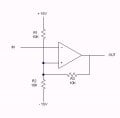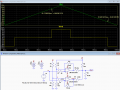# Setting an Inverting Schmitt Trigger (op amp)

#### clark.donaldson

Joined Aug 19, 2019
27
Hello -

I have a waveform that goes from -10v to 10v. When it reaches 10v, I would like the Schmitt Trigger to output -10v. When the waveform reaches -10v, I would like the Schmitt Trigger to output 10v.

I believe the attached schematic is the standard circuit for doing this. But I am having trouble understanding how. It seems that Schmitt Triggers seem to be "tripped" at the ground voltage, instead of near either rail.

Is there a better op-amp or comparator circuit for this job?

#### KeithWalker

Joined Jul 10, 2017
595
Hello -

I have a waveform that goes from -10v to 10v. When it reaches 10v, I would like the Schmitt Trigger to output -10v. When the waveform reaches -10v, I would like the Schmitt Trigger to output 10v.

I believe the attached schematic is the standard circuit for doing this. But I am having trouble understanding how. It seems that Schmitt Triggers seem to be "tripped" at the ground voltage, instead of near either rail.

Is there a better op-amp or comparator circuit for this job?
You need to add hysteresis to trigger at + and - 10v rather than at one set voltage.

#### ericgibbs

Joined Jan 29, 2010
9,305
hi clark,
What are the rise and fall rates of the +/-10V inputs.. ie: slow or square wave.?
What are the supply voltages to the OPA.?
E

#### clark.donaldson

Joined Aug 19, 2019
27
What are the rise and fall rates of the +/-10V inputs.. ie: slow or square wave.?
What are the supply voltages to the OPA.?
E
The rise and fall rates are variable. Ideally between 0.01 and 100 cps.
The supply voltage is +/-15v.

You need to add hysteresis to trigger at + and - 10v rather than at one set voltage.
Can you help me understand how to do that?

#### ericgibbs

Joined Jan 29, 2010
9,305
hi clark,
Are you saying Ideally between 0.01 and 100 cps, does this mean sinusoidal.?

We need to know the shape of the input waveforms.
E

#### crutschow

Joined Mar 14, 2008
24,062
What's the tolerance on the plus and minus 10V output levels?

#### clark.donaldson

Joined Aug 19, 2019
27
hi clark,
Are you saying Ideally between 0.01 and 100 cps, does this mean sinusoidal.?

We need to know the shape of the input waveforms.
E
The goal is for somewhat linear ramps that rise and fall at adjustable rates.

#### clark.donaldson

Joined Aug 19, 2019
27
What's the tolerance on the plus and minus 10V output levels?
It can be pretty flexible, if +/- 10v is problematic.

#### crutschow

Joined Mar 14, 2008
24,062
It can be pretty flexible, if +/- 10v is problematic.
It's not problematic, I just needed to need how close it should be to 10V.

#### clark.donaldson

Joined Aug 19, 2019
27
It's not problematic, I just needed to need how close it should be to 10V.
Well, I can add another op-amp to amplify things after the fact if a different value is better. But +/- 0.5v tolerance would be "good enough."

#### KeithWalker

Joined Jul 10, 2017
595
•clark.donaldson

#### KeithWalker

Joined Jul 10, 2017
595
Here is a simplified explanation for you. Look at the circuit below:
When the input is at 0 volts, the comparator has two stable states.
If the output is at +15 volts, the reference on the + input will be +10 volts and it will remain that way until the - input exceeds +10 volts. Then the voltage on the output will become -15 volts. The reference voltage on the + input will change to -10 volts and it will be stable until the - input is lower than -10 volts.
This is a simplified explanation because the output will not switch between +/- supply voltage, but to something a little less. You will have to change
the value of R3 to suit the characteristics of the comparator you are using.•clark.donaldson

#### crutschow

Joined Mar 14, 2008
24,062
Here's a modified window comparator circuit using an LM339 or 393, that I think does what you want.
The LTspice simulation shows that when the input is below -10V the output is -10V, when above +10V it's +10V, and it's 0V otherwise.
It uses resistive dividers from the ±15V supplies to generate the trip point and the output voltage levels, so those levels will vary with any change in the 15V supply voltages.
The feedback to the (+) inputs provides about 50mV of hysteresis to minimize any oscillations at the trip point.
If you want a lower output impedance than the resistors provide (13.3kΩ max), you could add a non-inverting buffer op amp.Last edited:
•clark.donaldson

#### clark.donaldson

Joined Aug 19, 2019
27
Hi all -
I tested the simple Schmitt trigger circuit first, since it was quick to mock up. I haven't put it through all the paces yet, but it seems to work fine. Is there any reason that a window comparator circuit would be necessary instead?

Also, could you give me the equation for the threshold math? If it's (R2/R1+R2) * V - I'm not sure how it produces 10v.

#### crutschow

Joined Mar 14, 2008
24,062
Is there any reason that a window comparator circuit would be necessary instead?
My understanding is, you want two input threshold levels with three different output voltages.
If you can do that with your circuit, then go for it.could you give me the equation for the threshold math? If it's (R2/R1+R2) * V - I'm not sure how it produces 10v.
Just plug in the numbers.
That's 10k / (5k+10k) * 15v = 10 / 15 * 15 = 10V.
Why didn't the math work for you?

Last edited:
•clark.donaldson

#### clark.donaldson

Joined Aug 19, 2019
27
My understanding is, you want two input threshold levels with three different output voltages.
Oh no, I only wanted two output voltages. When the voltage goes above 10v, I want -10v output, when it goes below -10v, I wanted 10v outputted.

Why didn't the math work for you?
The Schmitt circuit above used all 10k resistors.

#### crutschow

Joined Mar 14, 2008
24,062
Oh no, I only wanted two output voltages. When the voltage goes above 10v, I want -10v output, when it goes below -10v, I wanted 10v outputted.
And what output do you want when it's between those two voltages?
The Schmitt circuit above used all 10k resistors.
Okay.

#### KeithWalker

Joined Jul 10, 2017
595
Oh no, I only wanted two output voltages. When the voltage goes above 10v, I want -10v output, when it goes below -10v, I wanted 10v outputted.

The Schmitt circuit above used all 10k resistors.
When the output of the comparator is high, R3 is in parallel with R1 and when the output is low, R3 is in parallel with R2.
To get a hysteresis of +/- 10 volts, R3 = 2.5 Kohms.

Last edited:

#### clark.donaldson

Joined Aug 19, 2019
27
It seems that the "trip" point, or threshold is still roughly around the 0v/ground point. Is that accurate?

#### ericgibbs

Joined Jan 29, 2010
9,305
It seems that the "trip" point, or threshold is still roughly around the 0v/ground point. Is that accurate?
hi,
The trip points are at +/-10V , not around 0V.
Refer post #13, observe the rising/falling input Ramp voltage.
E